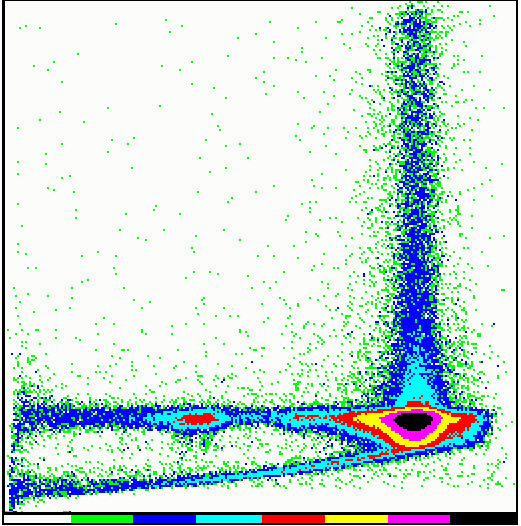[continues from 4.11.3]

## 4.12 Burst Length corrections

A first correction for BL selection to the total efficiency is taken into account in modelling the scintillation region. A further term has to be introduced to consider the loss of efficiency due to the high BL threshold. The factor b(E,B1,B2) which enters in the response matrix calculation is a correction factor to the efficiency which depends on the Burst Length limits B1 and B2 used during the accumulation. This is currently modelled by a function only for one fixed optimal range B1,B2: such range (27-60 for M1 and M3, 25-55 for M2) is the one which must be used when generating the response matrix.

```
b(E,B1,B2) = 1				if E<F1

b(E,B1,B2) = F2 + F3 exp[(E-F1)/F4] 	otherwise

```

The relevant coefficients F (together with B1 and B2) are kept in files m{1,2,3}_blsel.coeff.

Fig. 4.12-I : MECS BL selection coefficients vs energy (click on figure to expand Y scale)

The following figure shows a typical energy-burst length image (number of counts vs PHA channel on X-axis, and BL channels on Y-axis, both ranging 0-255).Fig. 4.12-II : example of E-BL image for an exposure taken on the ground (MPE Panter facility) This particular exposure is in Cu light (8.4 keV). The X and Y scale are in PHA and BL channels (both ranging 0-255).

The horizontal strip corresponds to the normal BL range, and contains the continuum underlying the line, the line itself (i.e. the events numbered as 1-2 in the event interaction figure), and the escape peak (fluorescence events numbered as 4). The vertical strip is due to events indicated with number 3 (recombination of primary and fluorescence at long BL). The lower oblique branch is due to events (not shown in quoted figure) absorbed in the scintillation region with a lower penetration depth (they produce a reduced amount of UV light, resulting in a shorter BL and a wrong PHA value).